# ACT Math : How to order decimals from least to greatest or from greatest to least

## Example Questions

### Example Question #1 : How To Order Decimals From Least To Greatest Or From Greatest To Least

Order the following decimals from greatest to least:

0.999, 0.909, 0.990

0.999, 0.909, 0.990

0.999, 0.990, 0.909

0.990, 0.999, 0.909

0.909, 0.999, 0.990

0.909, 0.990, 0.999

0.999, 0.990, 0.909

Explanation:

The greatest is 0.999 (999/1000), then 0.990 (990/1000), finally 0.909 (909/1000).

0.999 > 0.990 > 0.909

### Example Question #2 : How To Order Decimals From Least To Greatest Or From Greatest To Least

Place the following numbers in order from smallest to greatest: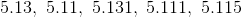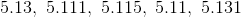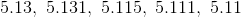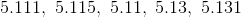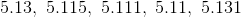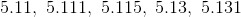Explanation:

When comparing decimals, move left to right, comparing the size of the tenths, then the hundredths, then the thousandths, etc….

### Example Question #3 : How To Order Decimals From Least To Greatest Or From Greatest To Least

Arrange the following from least to greatest: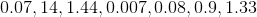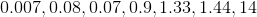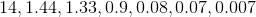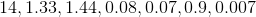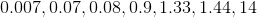Explanation:

From least to greatest:

0.007 (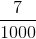), 0.07 (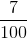), 0.08 (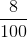), 0.9 (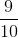), 1.33 (and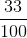), 1.44 (and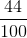), 14

### Example Question #1 : How To Order Decimals From Least To Greatest Or From Greatest To Least

Put the following in order of least to greatest: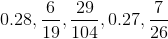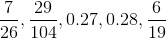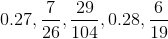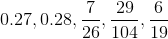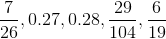Explanation:

Begin by converting each fraction into a decimal: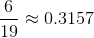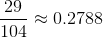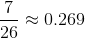Now we can put the decimals in order:

0.269, 0.27, 0.2788, 0.28, 0.3157

or: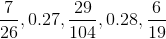### Example Question #4 : How To Order Decimals From Least To Greatest Or From Greatest To Least

Order the following decimals from greatest to least: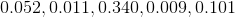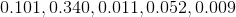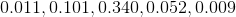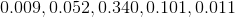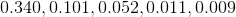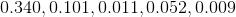Explanation:

With decimals, the larger number has a value in the larger spot (anything with a nonzero value in the tenths, is larger than any number with a 0 in the tenths and a digit in the hundredths, and so on). Thus the correct ordering starts with the decimal with the largest digit in the tenth spot, or .340.

After that there is only one with a nonzero value in the tenths spot, so .101 comes next.

Then there are only two that have nonzero values in the hundreths spot, and since 5 is larger than 1 the next two are .052 and then .011.

Finally, the smallest number is the one with zeroes in the tenths and hundreths spot so .009 is the smallest.

Thus resulting in,### Example Question #5 : How To Order Decimals From Least To Greatest Or From Greatest To Least

Order the following from least to greatest: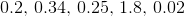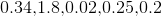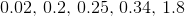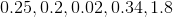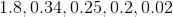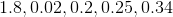Explanation: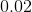, equivalent to two-hundredths, is the least out of the collection.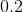, equivalent to twenty-hundredths, is the next highest.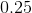, equivalent to twenty-five hundredths, is the next highest.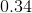, equivalent to thirty-four hundredths, is the next highest.

Finally, the highest number is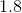, equivalent to one hundred and eighty hundredths.